Previous: 10. Evaluation of Quantum Up: 10. Evaluation of Quantum Next: 10.1.1 I-V curves

# 10.1 Comparison of Simulation Results

With regard to coherent transport both the quantum transmitting boundary method and the Wigner function method try to implement the same physical model: Particles are injected from the electrodes with a fixed distribution. Outgoing particles can leave the device without being reflected and are absorbed at the boundaries.

In this section we do not try to replicate any of the advanced'' simulations found in the literature. Instead we will concentrate on a much more down-to-earth question: Does the Wigner standard discretization accurately solve the physical model it describes? For ballistic transport we compare results from the Wigner method with results from the quantum transmitting boundary method.

For a numerical exemplification we present simulation results for a resonant tunneling diode with the following specification: The RTD model consists of a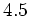nm-wide GaAs quantum well and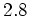nm-wide AlGaAs barrier layers. The GaAs electrode layers of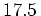nm are included on each side of the device. The conduction band discontinuity is taken to be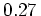eV. We use a constant effective mass of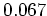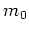. The doping density in the GaAs electrodes is given as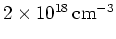and is used to calculate the distribution of the incoming carriers. Scattering processes are neglected and all calculations are performed at a room temperature (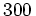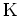).

We assume a flat band model where the voltage drops linearly across the barrier and well regions. This non self-consistent model is chosen for ease of numerical comparison.

For the finite difference Wigner solver we use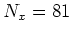and. These are typical values for the mesh size used in the literature. We use Frensley's standard discretization. No relaxation time scattering is included.

For the Schrödinger solver we use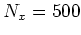and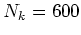. The mesh in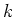is adapted to resolve the resonances accurately. The choice of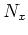guarantees that resolution is at least 20 points per wave length. In this way a numerically accurate solution is obtained.

SubsectionsPrevious: 10. Evaluation of Quantum Up: 10. Evaluation of Quantum Next: 10.1.1 I-V curves